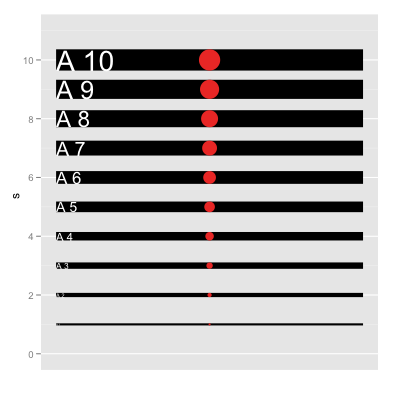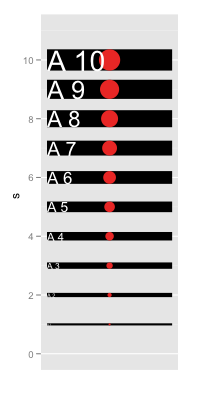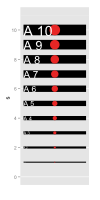## ggplot2 Quick Reference: size

Most geoms have a "size" parameter. For points, the size corresponds to their diameter. For lines, the size corresponds to their width. For text, the size corresponds to the height of their font.

Legal size values are any numbers greater than or equal to 0. For most geoms, the default size is 0.5.

The size can be set to a constant value or it can be mapped via a scale.

### Setting to constant value

To set the size to a constant value, use the size geom parameter (e.g., geom_point(data=d, mapping=aes(x=x, y=y), size=5) sets the size of all points in the layer to 5.

### Mapping with scale_size_identity

The scale_size_identity scale can be used to pass through any legal size value (its mapping is the identity function, and thus it does not change anything).```d=data.frame(s=0:10)
ggplot(data=d) +
scale_x_continuous(name="", limits=c(-1,1), breaks=NA) +
scale_y_continuous(limits=c(0,11)) +
scale_size_identity() +
geom_segment(mapping=aes(x=-1, y=s, xend=1, yend=s, size=s))+
geom_point(mapping=aes(x=0, y=s, size=s), color="red")+
geom_text(mapping=aes(x=-1, y=s, label=paste("A", s), size=s), hjust=0, color="white")```

### Note

The size does not relate to the coordinate system or the position scales of the plot in any way! The size (when using an identity scale or when setting it directly) is an absolute measure. A size of 1 corresponds to approximately 0.75 mm (or a font size of 3.75).

When rendering to bitmaps, the size in number of pixels thus depends on the resolution (e.g., `png(..., res=72)`) of the output device (the PNG device uses 72 pixels per inch, the resolution of an old-fashioned computer screen, as its default resolution).

In the example below, the left bitmap was created with `png(..., width=200, height=400, res=72)`, the right bitmap with `png(..., width=100, height=200, res=36)` and zoomed in HTML (so the second one has the same size, but only half the resolution, on the screen).